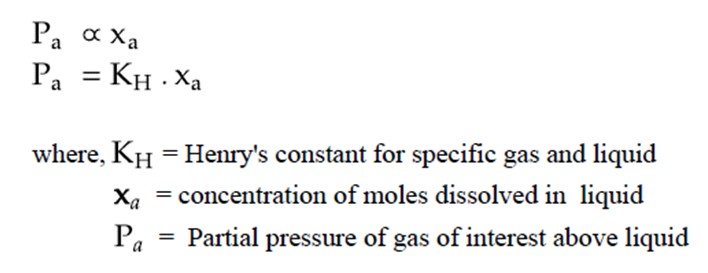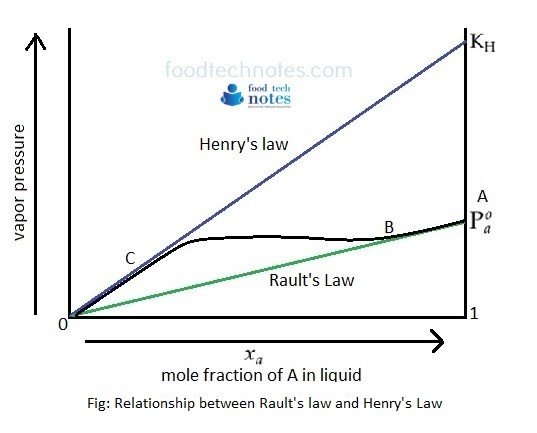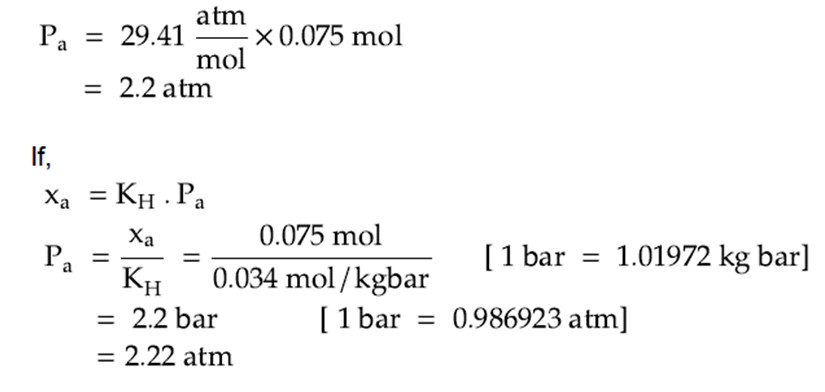# Henry’s Law

Henry’s Law states that partial pressure on component on a solution is directly proportional to mole fraction in liquid.Rault’s law is essentially a special case of Henry Law where constant becomes vapor pressure of pure component. Henry’s law is applicable for very dilute solution. It tells about how gas is dissolved in liquid. For example, concentration of gas (CO2) in cold drink cans can be calculated by using Henry’s law. Thus Henry’s law is often used to describe solubility of gas in liquid.

When the partial pressure of one component A is plotted against mole fraction of component A which is not ideal, the relationship between Rault’s Law and Henrys law is explained by following diagram.In figure, mixture follows Henry’s law from 0 to C and follows Rault’s Law from B to A.

#### Numerical Practice: If the concentration of CO2 dissolved in sparkling water in a closed soda can is 0.075 mol, what is the partial pressure of CO2 (g) in can at 25°C.